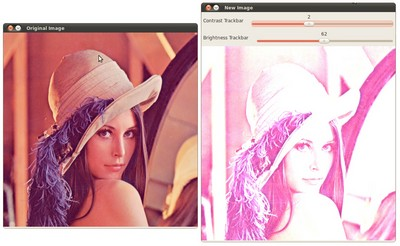# Adding a Trackbar to our applications!¶

• In the previous tutorials (about linear blending and the brightness and contrast adjustments) you might have noted that we needed to give some input to our programs, such as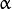and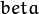. We accomplished that by entering this data using the Terminal

• Well, it is time to use some fancy GUI tools. OpenCV provides some GUI utilities (highgui.h) for you. An example of this is a Trackbar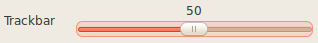• In this tutorial we will just modify our two previous programs so that they get the input information from the trackbar.

## Goals¶

In this tutorial you will learn how to:

## Code¶

Let’s modify the program made in the tutorial Adding (blending) two images using OpenCV. We will let the user enter thevalue by using the Trackbar.

#include <cv.h>
#include <highgui.h>

using namespace cv;

/// Global Variables
const int alpha_slider_max = 100;
int alpha_slider;
double alpha;
double beta;

/// Matrices to store images
Mat src1;
Mat src2;
Mat dst;

/**
* @function on_trackbar
* @brief Callback for trackbar
*/
void on_trackbar( int, void* )
{
alpha = (double) alpha_slider/alpha_slider_max ;
beta = ( 1.0 - alpha );

addWeighted( src1, alpha, src2, beta, 0.0, dst);

imshow( "Linear Blend", dst );
}

int main( int argc, char** argv )
{
/// Read image ( same size, same type )

if( !src1.data ) { printf("Error loading src1 \n"); return -1; }
if( !src2.data ) { printf("Error loading src2 \n"); return -1; }

/// Initialize values
alpha_slider = 0;

/// Create Windows
namedWindow("Linear Blend", 1);

/// Create Trackbars
char TrackbarName;
sprintf( TrackbarName, "Alpha x %d", alpha_slider_max );

createTrackbar( TrackbarName, "Linear Blend", &alpha_slider, alpha_slider_max, on_trackbar );

/// Show some stuff
on_trackbar( alpha_slider, 0 );

/// Wait until user press some key
waitKey(0);
return 0;
}


## Explanation¶

We only analyze the code that is related to Trackbar:

1. First, we load 02 images, which are going to be blended.

src1 = imread("../../images/LinuxLogo.jpg");

2. To create a trackbar, first we have to create the window in which it is going to be located. So:

namedWindow("Linear Blend", 1);

3. Now we can create the Trackbar:

createTrackbar( TrackbarName, "Linear Blend", &alpha_slider, alpha_slider_max, on_trackbar );


Note the following:

• Our Trackbar has a label TrackbarName
• The Trackbar is located in the window named “Linear Blend”
• The Trackbar values will be in the range from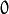to alpha_slider_max (the minimum limit is always zero).
• The numerical value of Trackbar is stored in alpha_slider
• Whenever the user moves the Trackbar, the callback function on_trackbar is called
4. Finally, we have to define the callback function on_trackbar

void on_trackbar( int, void* )
{
alpha = (double) alpha_slider/alpha_slider_max ;
beta = ( 1.0 - alpha );

addWeighted( src1, alpha, src2, beta, 0.0, dst);

imshow( "Linear Blend", dst );
}


Note that:

• We use the value of alpha_slider (integer) to get a double value for alpha.
• alpha_slider is updated each time the trackbar is displaced by the user.
• We define src1, src2, dist, alpha, alpha_slider and beta as global variables, so they can be used everywhere.

## Result¶

• Our program produces the following output: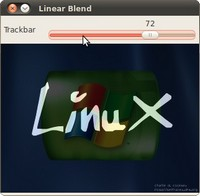• As a manner of practice, you can also add 02 trackbars for the program made in Changing the contrast and brightness of an image!. One trackbar to setand another for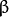. The output might look like: